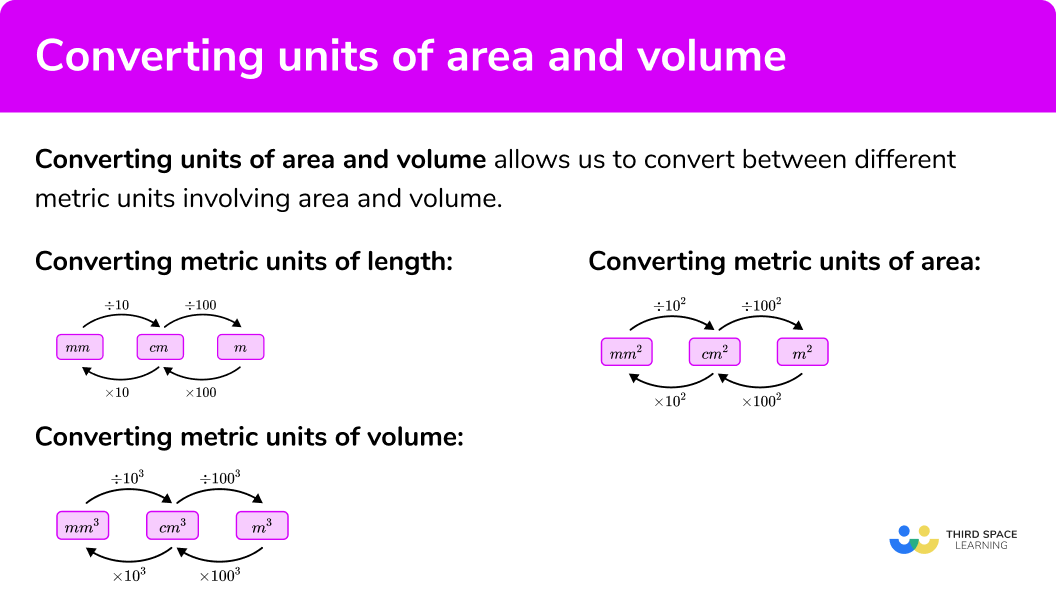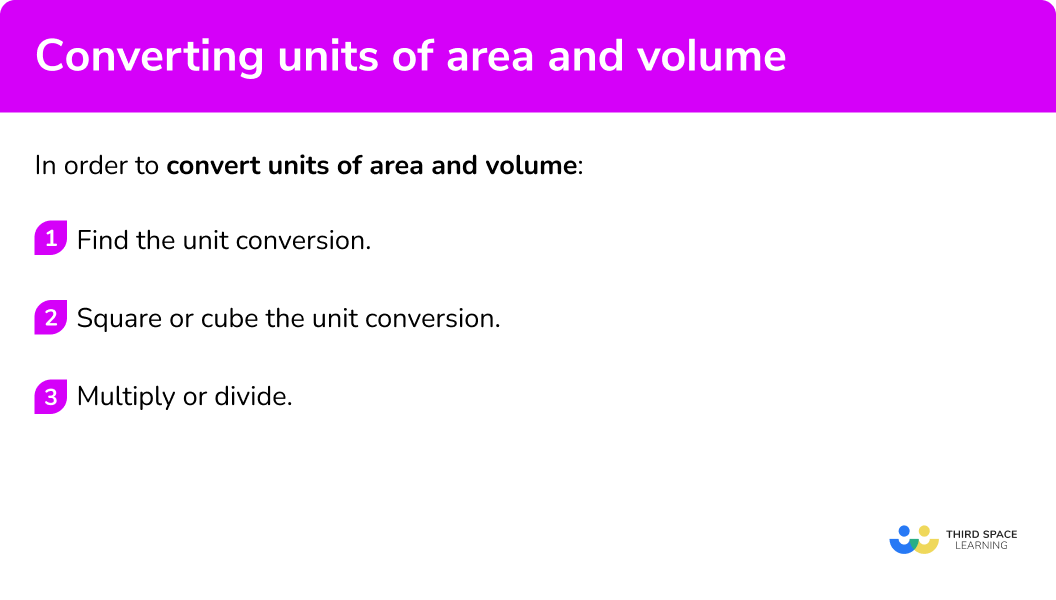GCSE Maths Ratio and Proportion Units Of Measurement

Converting Units Of Area And Volume

# Converting Units Of Area And Volume

Here we will learn about converting units of area and volume.

There are also worksheets based on Edexcel, AQA and OCR exam questions, along with further guidance on where to go next if you’re still stuck.

## What is converting units of area and volume?

Converting units of area and volume is converting between different units from the metric system involving area and volume.

To do this we need to be able to convert between different units of length, and then adapt them for area and volume.

### What is converting units of area and volume?### Units of length

We know that 1 \ m =100 \ cm and 1 \ cm =10 \ mm , so we can convert between these lengths:

Step-by-step guide: Converting metric units

We can then apply these to units of measurement for area and volume.

### Units of area

Let’s apply this to area:

We know that 1 \ cm = 10 \ mm

So, 1 \ cm^2 = 10 \times 10 =10^2 =100 \ mm^2

Similarly we know that 1 \ m = 100 \ cm

So, 1 \ m^2 = 100 \times 100 =100^2 =10 \ 000 \ cm^2

### Units of volume

Let’s apply this now to volume:

We know that 1 \ cm =10 \ mm

So, 1 \ cm^3 =10 \times 10 \times 10 =10^3 =1000 \ mm^3

Similarly we know that 1 \ m = 100 \ cm

So, 1 \ m^3 =100 \times 100 \times 100 =100^3 =1 \ 000 \ 000 \ cm^3

E.g.

Convert 7 \ m^2 to cm^2

7\times 100^2 =70\ 000

So, 7 \ m^2 = 70 \ 000 \ cm^2

## How to convert units of area and volume

In order to convert units of area and volume:

1. Find the unit conversion.
2. Square or cube the unit conversion.
3. Multiply or divide.

### How to convert units of area and volume## Related lessons on units of measurement

Converting units of area and volume is part of our series of lessons to support revision on units of measurement. You may find it helpful to start with the main units of measurement lesson for a summary of what to expect, or use the step by step guides below for further detail on individual topics. Other lessons in this series include:

## Converting units of area examples

### Example 1: converting square metres and square centimetres

Convert 3 \ m^2 to cm^2

1. Find the unit conversion.

The units involve metres and centimetres

1 \ m=100 \ cm

2Square or cube the unit conversion.

The question involves square units, so we need to square the unit conversion.

100^2 = 10\ 000

3Multiply or divide.

As we are going from larger units to smaller units we multiply.

3 \times 100^2 = 3 \times 10 \ 000=30 \ 000

So, \ 3 \ m^2 \ is \ 30 \ 000 \ cm^2

### Example 2: converting square metres and square centimetres

Convert 45 \ 000 \ cm^2 to m^2

The units involve metres and centimetres

1 \ m= 100 \ cm

The question involves square units, so we need to square the unit conversion.

100^2 =10 \ 000

As we are going from smaller units to larger units we divide.

45 \ 000 \div 100^2 =45 \ 000 \div 10 \ 000 =4.5

So, \ 45 \ 000 \ cm^2 \ is \ 4.5 \ m^2

### Example 3: converting square centimetres and square millimetres

Convert 6.1 \ cm^2 to mm^2

The units involve centimetres and millimetres

1 \ cm= 10 \ mm

The question involves square units, so we need to square the unit conversion.

10^2 =100

As we are going from larger units to smaller units we multiply.

6.1 \times 10^2 = 6.1 \times 100 =610

So, \ 6.1 \ cm^2 \ is \ 610 \ mm^2

### Example 4: converting square centimetres and square millimetres

Convert 7800 \ mm^2 to cm^2

The units involve centimetres and millimetres

1 \ cm= 10 \ mm

The question involves square units, so we need to square the unit conversion.

10^2 =100

As we are going from smaller units to larger units we divide.

7800 \div 10^2 =7800 \div 100 = 78

So, \ 7800 \ mm^2 \ is \ 78 \ cm^2

### Example 5: converting cubic centimetres and cubic millimetres

Convert 5 \ cm^3 to mm^3

The units involve centimetres and millimetres

1 \ cm = 10 \ mm

The question involves cubic units, so we need to cube the unit conversion.

10^3 =1000

As we are going from larger units to smaller units we multiply.

5 \times 10^3 =5 \times 1000=5000

So, \ 5 \ cm^3 \ is \ 5000 \ mm^3

### Example 6: converting cubic centimetres and cubic millimetres

Convert 68 \ 000 \ mm^3 to cm^3

The units involve centimetres and millimetres

1 \ cm = 10 \ mm

The question involves cubic units, so we need to cube the unit conversion.

10^3 = 1000

As we are going from smaller units to larger units we divide.

68 \ 000 \div 10^3 =68 000 \div 1000=68

So, \ 68 \ 000 \ mm^3 \ is \ 68 \ cm^3

### Common misconceptions

• Remember to square or cube the unit conversion

You need to remember to square the unit conversion for units of area.

You need to remember to cube the unit conversion for units of volume.

E.g. convert 3 \ m^2 to cm^2

This would be incorrect \; \color{red} ✘

3 \times 100=300

This would be correct \; \color{green} ✔

3 \times 100^2 =3 \times 10 \ 000 = 30 \ 000

3 \ m^2 =30 \ 000 \ cm^2

### Practice converting units of area questions

1. Convert: \ 5.6 \ m^2 to cm^2

5\ 600 \ cm^256\ 000 \ cm^2560 \ cm^2560\ 000 \ cm^2We need to multiply by 100^2 as we are converting m^2 to cm^2

5.6\times 100^2 = 5.6 \times 10\ 000 = 56\ 000

2. Convert: \ 840 \ 000 \ cm^2 to m^2

84\ 000 \ m^2840 \ m^28\ 400 \ m^284 \ m^2We need to divide by 100^2 as we are converting cm^2 to m^2

840\ 000\div 100^2 = 840\ 000\div 10\ 000 = 84

3. Convert: \ 94 \ cm^2 to mm^2

9.4 \ mm^2940 \ mm^29\ 400 \ mm^20.94 \ mm^2We need to multiply by 10^2 as we are converting cm^2 to mm^2

94\times 10^2 = 94\times 100 = 9 \ 400

4. Convert: \ 570 \ mm^2 to cm^2

5.7 \ cm^257 \ cm^20.57 \ cm^25\ 700 cm^2We need to divide by 10^2 as we are converting mm^2 to cm^2

570\div 10^2 = 570\div 100 = 5.7

5. Convert: \ 6.3 \ cm^3 to mm^3

63 \ mm^3630 \ mm^30.63 \ mm^36\ 300 \ mm^3We need to multiply by 10^3 as we are converting cm^3 to mm^3

6.3\times 10^3 = 6.3\times 1000 = 6\ 300

6. Convert: \ 9 \ 100 \ mm^3 to cm^3

91\ 000 \ cm^391 \ cm^39.1 \ cm^3910 \ cm^3We need to divide by 10^3 as we are converting mm^3 to cm^3

9 \ 100\div 10^3 = 9 \ 100\div 1000 = 9.1

### Converting units of area GCSE questions

1. Write 35 \ cm^3 in mm^3

(2 marks)

35\times 10^3

(1)

35 \ 000

(1)

2. Write 48 \ m^2 in cm^2

(2 marks)

48\times 100^2

(1)

480 \ 000

(1)

3. In the garden there is an area that needs covering with bark chippings.

The area is 210 \ 000 \ cm^2.

A bag of bark chippings covers 4 \ m^2.

How many bags of bark chippings are needed to be bought to cover the area?

(3 marks)

210\ 000\div 100^2=21

(1)

21\div 4=5.25

(1)

6 bags are needed to be bought.

(Rounding up as we can only buy full bags.)

(1)

## Learning checklist

You have now learned how to:

• Convert metric units of area
• Convert metric units of volume

## The next lessons are

### Did you know?

There are non-metric units of area and non-metric units of volume.

These are called imperial units.

• Imperial units of area
There are non-metric units that can be used for area.
E.g.
There are 12 inches in 1 foot.
If we wanted to convert between square inches and square feet we would use the multiplier 12^2
So 5 square feet would be 5 \times 12^2 square inches which is 720 square inches.
We can do a similar thing for square feet and square yards.
E.g.
There are 3 feet in 1 yard, so we would use 3^2 to convert between square feet and square yards.
This can be extended to the square mile.
E.g.
There are 1760 yards in 1 mile, so we would use 1760^2 to convert between square feet and square miles.

• Imperial units of volume
There are non-metric units that can be used for volume.
E.g.
There are 12 inches in 1 foot.
If we wanted to convert between cubic inches and cubic feet we would use the multiplier 12^3
So 4 cubic feet would be 4 \times 12^3 cubic inches which is 6912 cubic inches.
We can do similar for cubic feet and cubic yards.
E.g.
There are 3 feet in 1 yard, so we would use 3^3 to convert
between cubic feet and cubic yards.

## Still stuck?

Prepare your KS4 students for maths GCSEs success with Third Space Learning. Weekly online one to one GCSE maths revision lessons delivered by expert maths tutors.

Find out more about our GCSE maths tuition programme.